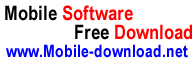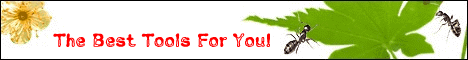Contact Us
 | Windows Mobile | Symbian | iPhone | Android OS | BlackBerry | Palm | Flash Lite | Web Applications |
 Hacker Tools » Download -> Symbian-> Nokia Series 60v2-> Graphics » Graph

 This Directory TOP18Acd SeePhotospyMMS-FX PixelateDS FogDS BumpMapDS RotScaleDS WobbleDS FireTunnelMMS-FX RotateMMS-FX LensSearch
 Name Detail# Graph

 File Size: 16KB Update Time: 2009-03-19 Developer: Description: It is written in 2005 by Alexander "Rogrog" Taboriskiy. The latest version of program has been tested at Nokia 6600, 6260, 6670, 3650, 3660, N-Gage, N-Gage QD, and it must work at any other Series60-based phone. THE MAIN FEATURES: - Plotting graphs of various functions - Viewing of it in any area - Opportunity of the task of parameter - Integrated scientific calculator - User-friendly intuitive interface SUPPORTED FUNCTIONS: Arithmetic: `+`, `-`, `*`, `/`, `^`: Y=X; Y=2*X; Y=5*X-1; With brackets and modules: : Y=abs (X); Y = (X+1) / (X-1); Y=abs (2*X^2+abs (X)-1); With trigonometrical functions: Y=sin (X); Y=cos (X); Y=tg (X); Y=ctg (X); With inverse trigonometrical functions: Y=arcsin (X); Y=arccos (X); Y=arctg (X); With logarithms: Y=ln (X); Y=exp (X); Y=log (X); Allocation of a integer / fractional part: Y=int (X); Y=frac (X); Download: Graph